# Basic signals

In the analysis of communication system, standard test signals play very important role. Such signals are used to check the performance of the system. Applying such signals at the system; the output is checked. Now depending on the input-output characteristic of that particular system; study of different properties of a system can be done. Some standard test signals are as follows:

• Delta or unit impulse function

• Unit step signal

• Unit ramp signal

• Exponential signal

• Sinusoidal signal

## Delta or unit impulse function:

• A discrete time unit impulse function is denoted by d(n). Its amplitude is 1 at n=0 and for all other values of n; its amplitude is zero.

d(n)= 1 for n=0

d(n)=0 for n?0

In the sequence form it can be represented as,

d(n) = {….,0,0,0,1,0,0,0….}
or d(n) = {1}

The graphical representation of delta function for D.T. signal  is as shown in figure below:

• A continuous time delta function is denoted by d(t). mathematically it is expressed as follows:

d(t)=1 for t=0

d(t)=0 for t?0

The graphical representation of delta function for C.T. signal is shown in figure below:

[caption id="" align="aligncenter" width="332"]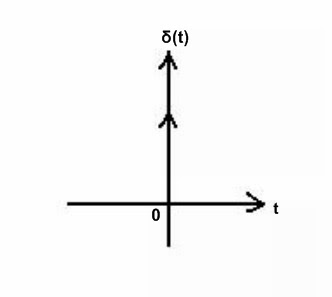Unit impulse function[/caption]

## Unit step signal:

Unit step signal means the signal has unit amplitude for positive axis and has zero amplitude for negative axis.

There are two types of unit step signal as follows:

1) Discrete time unit step signal

2) Continuous time unit step signal

Let us discuss these two types one by one:

## 1) Discrete time unit step signal

• A discrete time unit step signal is denoted by u(n). its value is unity (1) for all positive values of n. that means its value is one for n = 0. While for other values of n, its value is zero.

u(n)= 1 for  n = 0

u(n)= 0 for n < 0

In the form of sequence it can written as,

u(n) = {1,1,1,1,….}

Graphically it can be represented as follows:

## 2) Continuous time unit step signal

• A continuous type unit step signal is denoted by u(t). mathematically it can be expressed as,

u(t)= 1 for t = 0

u(t) = 0 for t < 0

it is shown in figure below:

## Unit ramp signal:

• A discrete time unit ramp signal is denoted by ur(n). its value increases linearly with sample number n. mathematically it is defined as,

Ur(n)= n for n = 0

Ur(n) = 0 for n < 0

From above equation, it is clear that the value of signal at a particular interval is equal to the number of interval at that instant. for example; for first interval signal has amplitude 1, for second it has amplitude 2, for third it is 3, and so on.

Graphically it is represented in figure below:

• A continuous time ramp type signal is denoted by r(t). mathematically it is expressed as,

r(t) = 1 for t = 0

r(t) = 0 for t < 0

From above equation, it is clear that the value of signal at a particular time is equal to the time at that instant. for example; for one second signal has amplitude 1, for two second it has amplitude 2, for third it is 3, and so on.

It is shown in figure below:

## Exponential signal:

In mathematics, the exponential function is the function ex, where e is the number (approximately 2.718281828) such that the function ex is its own derivative.

A discrete time exponential signal is expressed as,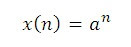Here ‘a’ is some real constant.

If ‘a’ is the complex number then x(n) is written as,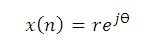Here ? denotes the phase. Now depending upon value of ? we have different cases:

case 1) : when a>1

Since the signal is exponentially growing; it is called rising exponential signal.

case 2) : when 0<a<1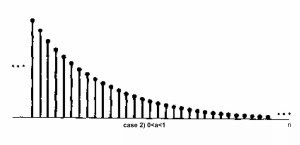Since the signal is exponentially decreases; it is called decaying exponential signal.

case 3) : when a<-1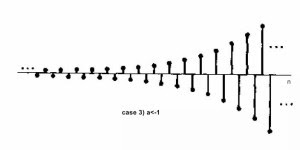As shown in figure above, both signals are growing hence it is called as double sided growing exponential signal.

case 4) : when -1<a<0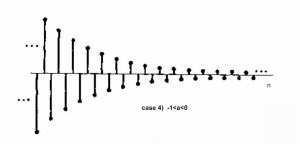As shown in figure above, both signals are decreases hence it is called as double sided decaying exponential signal.

• If we talk about continuous time exponential signals; everything is same as that of discrete time signals except it has continuous amplitude.

## Sinusoidal waveform:

A sinusoidal signal has the same shape as the graph of the sine function used in trigonometry. Sinusoidal signals are produced by rotating electrical machines such as dynamos and power station turbines and electrical energy is transmitted to the consumer in this form. In electronics, sine waves are among the most useful of all signals in testing circuits and analyzing system performance.

There are two types of sinusoidal waveforms:

1) Discrete time sinusoidal waveforms, and

2) Continuous time sinusoidal waveforms.

Let us discuss these waveforms one by one:

• Discrete time sinusoidal wave:

• Signals typically represent physical variables such as displacement, velocity, pressure, energy.

•  In most cases, we are concerned with variables that are time-dependent.

•  The discrete-time or digital time index is generally specified by tn = n T, where n is an integer and T is the sampling interval or period.

•  It is common to drop the explicit reference to T (or assume T = 1) and index discrete-time signals by the letter n.

• A discrete-time signal which is only dependent on time can be represented by x[n] for n = 0, 1, 2, ...

A discrete time sinusoidal waveform is denoted by,

x(n) = A sin (wn)

Here a = amplitude and w = angular frequency = 2pf

This waveform is as shown in figure below: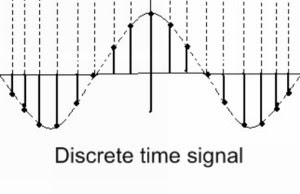Similarly a discrete time cosine waveform is expressed as,

x(n) = A cos (wn)

• Continuous time sinusoidal signals:

The sinusoidal signals include sine and cosine signals. They are as shown in figure below: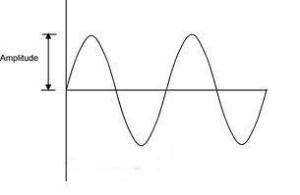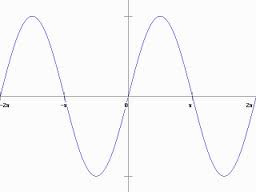Mathematically they can be expressed as follows:

A sine signal: x(t) = A sin (wt) = A sin (2pft)

A cosine signal: x(t) = A cos (wt) = A cos (2pft)